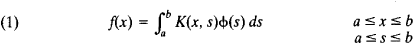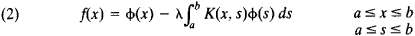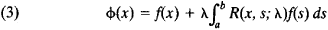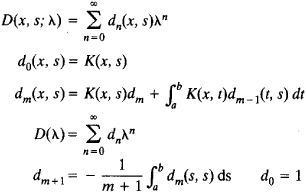# Fredholm Equation

## Fredholm Equation

The Fredholm equation of the first kind isThe Fredholm equation of the second kind isHere, K(x, s) is a given continuous function of x and s called the kernel of the equation, f(x) is a given function, ϕ(x) is the unknown function, and λ. is a parameter (seeINTEGRAL EQUATIONS).

Equations (1) and (2) were studied between 1900 and 1903 by E. Fredholm. The theory of Fredholm equations of the second kind is simpler, and these equations are more often used in applications. In the general case it is possible to obtain stable solutions of Fredholm equations of the first kind only with the help of special regularizing algorithms for the solution of incorrectly posed problems.

If λ is not an eigenvalue of equation (2), the equation has a unique continuous solution defined by the formulawhere, R(x, s; λ) = D(x, s; λ)/D(λ) is called the resolvent of equation (2). Here,References in periodicals archive ?
We consider the factor ([e.sup.i[tau]] - [e.sup.it]) in the expression ([z.sub.s](t) - [z.sub.s](t)), s = 0, 1 in order to separate the improper PV integral in the Fredholm equation of the second kind as follows [8, 9]:
We solve the integral Fredholm equation. This equation is well known and allows us to construct the conformal mapping of the given domain onto the unit disk .
Denoting the unknown function by u(t), we consider linear integral equations which involve an integral of the form [[integral].sup.b.sub.a]k(x,t)u(t)dt over a fixed interval is called a Fredholm equation, while if the upper limit is x, a variable, it is a Volterra equation.
To illustrate the efficiency of the proposed methods in studying the model equation (1), we solve four examples of Fredholm equations by the method of solution above.
Which is a Fredholm equation of the second kind with the Kernel K a given function on the square G = [a,b] x [a,b].
Currently, a variety of new numerical methods were proposed, for instance, the combination method of Galerkin and collocation with Haar wavelet function for the first kind of linear Fredholm equation , the Galerkin method with Daubechies wavelet for the second kind of linear Volterra equations , however, the calculation error of wavelet methods is relatively large.
Paper  shows that if the series converges its sum is the solution of the Fredholm equation. The same theorem for Volterra equation was proven in paper .
On the complexity of the problem of finding the solutions of Fredholm equations of the second kind with smooth kernels.
This equation is classified as a linear system of two-dimensional singular linear Fredholm equations of the second kind and is given by 

Site: Follow: Share:
Open / Close